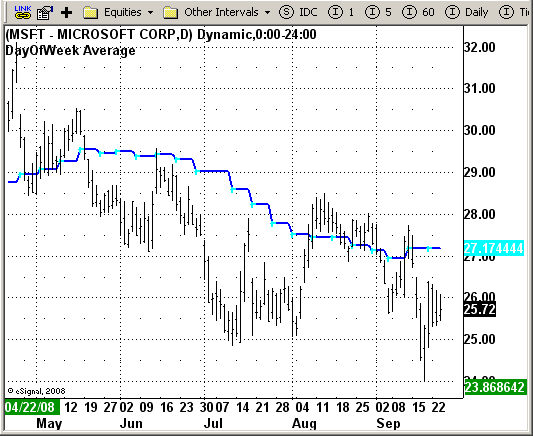# Day Of Week Average

ICE Data Services -

DayOfWeekAverage.efs                                                                                                                                              EFSLibrary - Discussion Board

File Name: DayOfWeekAverage.efs

Description:
Day Of Week Average

Formula Parameters:
Length: 13
Day Of Week: 5
The Day of the week to be Averaged
(1 = Mon, 2 = Tues, 3.....)
Smooth: 0
Defines a Raw or Smoothed Average
(0 = Smooth, 1 = Raw)
Price Data To Use: High

Notes:
This indicator plots the Moving Average of a specified price of a user
specified day of the week. The Study also plots a dot on the day of the
week that is being Averaged. This indicator is one of the best for measuring
the price change depending on the day of the week.

DayOfWeekAverage.efsEFS Code:

```/*********************************Provided By: eSignal (Copyright c eSignal),
This sample eSignal Formula Script (EFS) is for educational purposes only
and may be modified and saved under a new file name.
eSignal is not responsible for the functionality once modified.
eSignal reserves the right to modify and overwrite this EFS file with each new release.
Description: DayOfWeek AverageVersion: 1.0 09/23/2008Notes:
This indicator plots the Moving Average of a specified price of a user specified day of the week.
The Study also plots a dot on the day of the week that is being Averaged.
This indicator is one of the best for measuring the price change depending on the day of the week.
Formula Parameters: Default: * Length 13 * Day Of Week 5 The Day of the week to be Averaged
(1 = Mon, 2 = Tues, 3.....) * Smooth 0 Defines a Raw or Smoothed Average (0 = Smooth, 1 = Raw) * Price Data To Use High
**********************************/

var fpArray = new Array();
var bInit = false;

function preMain() {
setPriceStudy(true);
setStudyTitle("DayOfWeek Average");
setCursorLabelName("DOWAvg", 0);
setCursorLabelName("DayOWk", 1);
setDefaultBarFgColor(Color.blue, 0);
setDefaultBarFgColor(Color.cyan, 1);
setDefaultBarThickness(2, 0);
setDefaultBarThickness(2, 1);
setPlotType(PLOTTYPE_LINE, 0);
setPlotType(PLOTTYPE_DOT, 1);
var x = 0;
fpArray[x] = new FunctionParameter("Length", FunctionParameter.NUMBER);
with (fpArray[x++]) {
setLowerLimit(1);
setDefault(9);
}
fpArray[x] = new FunctionParameter("DayOfWeek", FunctionParameter.NUMBER);
with (fpArray[x++]) {
setName("Day Of Week (Mon=1, Tue=2. etc)");
setLowerLimit(1);
setDefault(5);
}
fpArray[x] = new FunctionParameter("Price", FunctionParameter.STRING);
with (fpArray[x++]) {
setName("Price Data To Use");
setDefault("high");
}
}
var DOWA = null;
var xMyPrice = null;
var DayOWkAvg = 0;
var DayOWkAvg_1 = 0;

function main(Length, Price, DayOfWeek) {
if (Price == null) Price = "high";
if (DayOfWeek == null) DayOfWeek = 5;
if (Length == null) Length = 9;
var Plot1 = null;
var Plot2 = null;
var nBar = getBarState() == BARSTATE_NEWBAR;
if (bInit == false) {
xMyPrice = eval(Price)();
bInit = true;
}
if (DOWA == null) {
DOWA = new Array(Length);
for (i = 0; i < Length; i++) {
DOWA[i] = 0;
}
}
var WeekAvg = 0;
var Date = getValue("Time", 0);
if (Date.getDay() == DayOfWeek && nBar) {
for (i = Length - 1; i > 0; i--) {
DOWA[i] = DOWA[i - 1];
}
DOWA = xMyPrice.getValue(0);
}
if (DOWA[Length - 1] != 0 && nBar) {
for (i = 0; i < Length; i++) {
WeekAvg += DOWA[i];
}
DayOWkAvg = WeekAvg / Length;
}
if (DayOWkAvg != 0) {
Plot1 = DayOWkAvg;
if (Date.getDay() == DayOfWeek) Plot2 = DayOWkAvg;
}
return new Array(Plot1, Plot2);
}
```